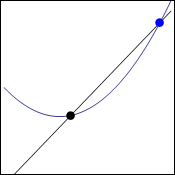# Quick point about equations and graphs

Hi,
mliae asked me to make some simpler post so she understands this post. So here it comes, she said that it was long since she used equations:

Well equation is something like this:

x=1

That is quite clear. Of course you can have very complicated equation with many “unknowns” which are usually noted as letters, x for example. All thats easy and it says that something (x) is equal to one, it has the value of 1.

We can manipulate these equations if we abide one rule: both sides (from left and right of equal sign) have to be manipulated. If we add one we have to do it on both sides:

x+1=1+1     —>    x+1=2

Easy. We can substitute in equations if we work with more:

a=b+42
2a+3=x

2(b+42)+3=x    (“a” was substitued by b+42 because that is what it equals to.)

Now in the post that is our concern we used this equation:To get here we have to use graph but I did not stop on that very much so I will go through it again.

Points on graph have two coordinates. This is because graph has 2 dimensions. These coordinates are usually called x and y and they are noted like this:

(x;y)

x says how much the point is to the left or right and y says how up or down (or closer/ further)

We had two points there on the graph:Now we will take the blue point as stacionary of course but since we are working in general and not with specific numbers, it should not matter.

So black point has coordinates x and y (x;y)

Since we want it to be general we will left it like this except the y. This is because we are going to derive functions and in those y=f(x) which means that y coordinate of the point is f(x). That is the notation that is used. We are working with the function f that gave coordinate y to our black point.

black_point(x;f(x))
blue_point(x+h;f(x+h))

The h should be clear from last time. It is the distance from the black point. Then when you insert this into the “slope” equation which I talked about here, you will get what you want.

Dragallur

Feel free to ask for more clarification.

## 5 thoughts on “Quick point about equations and graphs”

1.kristinakoti says:

Yes, it is very easy indeed. Derivation is how fast blue dot meets black dot 😀

Liked by 1 person

•Dragallur says:

😀

Liked by 1 person

2.mliae says:

Hey, thanks for stupiding it down for me 😉 I got it now! 😀

Liked by 1 person

•Dragallur says:

Great to hear it!

Liked by 1 person

•mliae says:

😀

Liked by 1 person